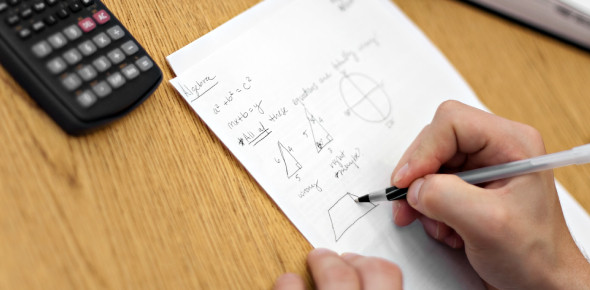# Maths Quiz On Similar Triangles

10 Questions | Attempts: 4890SettingsAre you a math wizard? Play this knowledgeable quiz on similar triangles to gauge your Maths knowledge. The quiz contains questions ranging from easy, medium, and hard levels that will test your conceptual understanding and help you increase your knowledge base. Triangles are one of the low-hanging fruit in any exam mastery over them can fetch you easy marks. If you like the quiz, share it with your friends and family. All the best!.

• 1.
Which of the following triangles are always similar?
• A.

Right triangle

• B.

Obtuse triangle

• C.

Equilateral triangle

• D.

Isoceles triangle

• 2.
The sides of a triangle are 7, 10, and 12.  Find the length of the longest side of a similar triangle whose shortest side is 21.
• A.

12

• B.

21

• C.

30

• D.

36

• 3.
In triangle ABC, angle A = 90º and angle B = 25º.  In triangle DEF, angle E = 25º and angle F = 65º.  Are the triangles similar?
• A.

True

• B.

False

• 4.
Two triangles are similar.  If the ratio of the perimeters is 5 : 3, find the ratio of the corresponding sides.
• A.

5 : 3

• B.

15 : 9

• C.

25 : 9

• D.

1:8

• 5.
Find the unknown length x in the following figure.
• 6.
Which of the following conditions are NOT sufficient to identify a pair of congruent triangles?
• A.

Three pairs of corresponding sides are proportional (SSS).

• B.

Two pairs of corresponding sides are proportional and their included angles are equal (SAS).

• C.

Two pairs of corresponding sides are proportional and one pair of non-included angles are equal (SSA).

• D.

Two pairs of angles are equal (AA).

• 7.
What is true of angles of similar triangles?
• A.

Opposite angles are congruent.

• B.

Corresponding angles are congurent.

• C.

Corresponding angles are 90 degrees.

• D.

Corresponding angles are parallel

• 8.
Area of an equilateral triangle with side length a is equal to:
• A.

√3/5a

• B.

√3/4a

• C.

√3/4 a2

• D.

√2/3 a

• 9.
If the perimeter of a triangle is 100 cm and the length of the two sides are 30 cm and 40 cm, the length of the third side will be:
• A.

30

• B.

45

• C.

24

• D.

16

• 10.
The height of an equilateral triangle of side 5 cm is:
• A.

5 cm

• B.

6.66 cm

• C.

4.33 cm

• D.

2.44 cm

## Related TopicsBack to top
×

Wait!
Here's an interesting quiz for you.